## Example Questions

### Example Question #161 : Arithmetic

What are three consecutive odd integers whose sum equals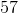?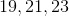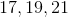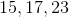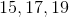Explanation:

Set up the equation,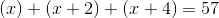.

Simplify to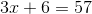and solve for x to find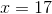.

Therefore the 3 consecutive odd integrers are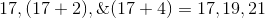.

### Example Question #162 : Arithmetic

Assumeandare both odd whole numbers and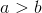.

What is a possible solution for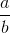?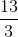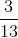Explanation:

The two requirements for this problem are that bothandmust be odd, and that. The only answer that fits both of these is. The other answers show either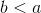oris an even number.

### Example Question #163 : Arithmetic

Solve for: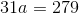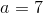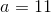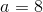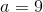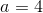Explanation:

To solve, divide both sides of the equation by: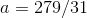As a check, if you divide an odd number by another odd number, your result should be odd.

### Example Question #164 : Arithmetic

Solve for: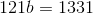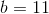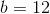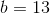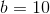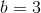Explanation:

To solve, isolate your variable by dividing both sides of the equation by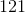: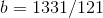### Example Question #165 : Arithmetic

Solve for: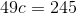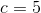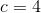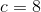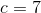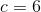Explanation:

To solve, first isolate your variable by dividing both sides of the equation by: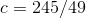As a check, if you divide an odd number by another odd number, your result should be odd.

### Example Question #101 : Integers

Solve for: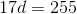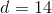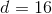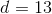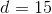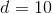Explanation:

To solve, divide both sides of the equation by: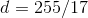As a check, if you divide an odd number by another odd number, your result should be odd.